If there is one prayer that you should pray/sing every day and every hour, it is the LORD's prayer (Our FATHER in Heaven prayer)
It is the most powerful prayer. A pure heart, a clean mind, and a clear conscience is necessary for it.
- Samuel Dominic Chukwuemeka

For in GOD we live, and move, and have our being. - Acts 17:28

The Joy of a Teacher is the Success of his Students. - Samuel Dominic Chukwuemeka# R and RStudio for Statistics

As at today: 06/26/2023;(3.) It leads to another website: https://cran.rstudio.com/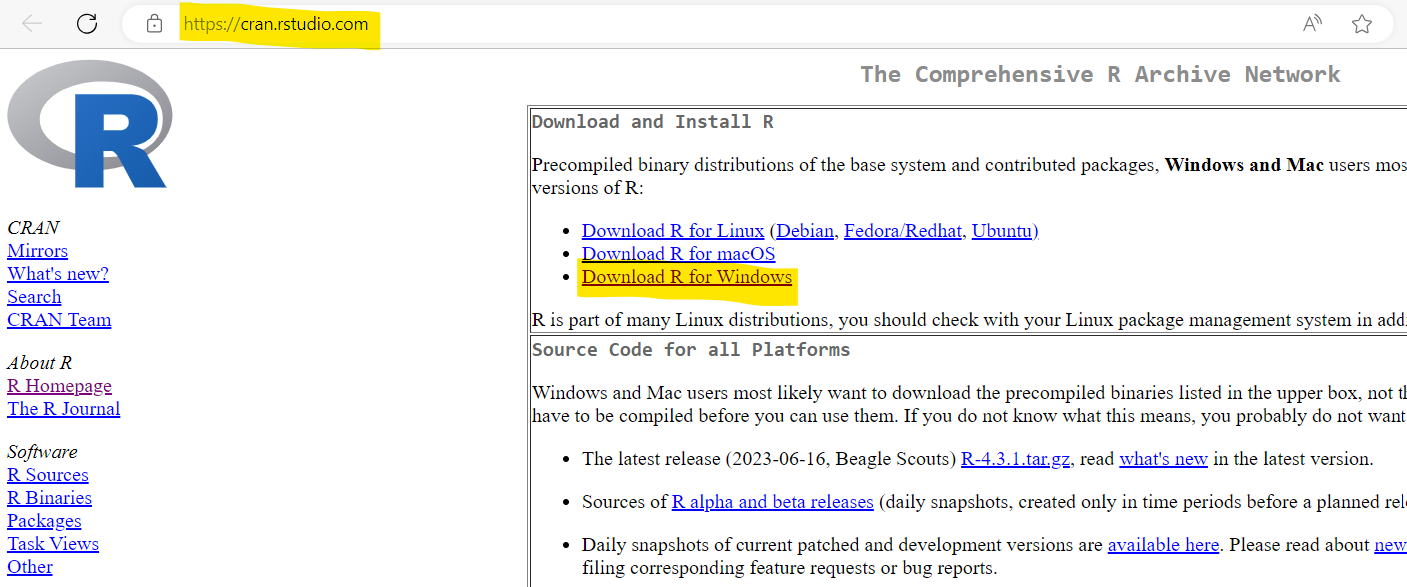(5.) Step 3: Click: base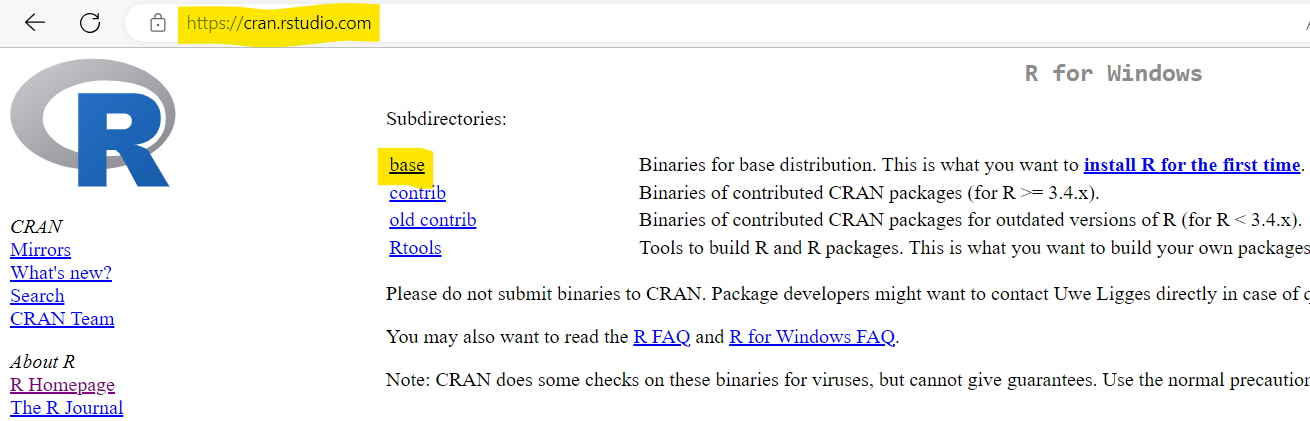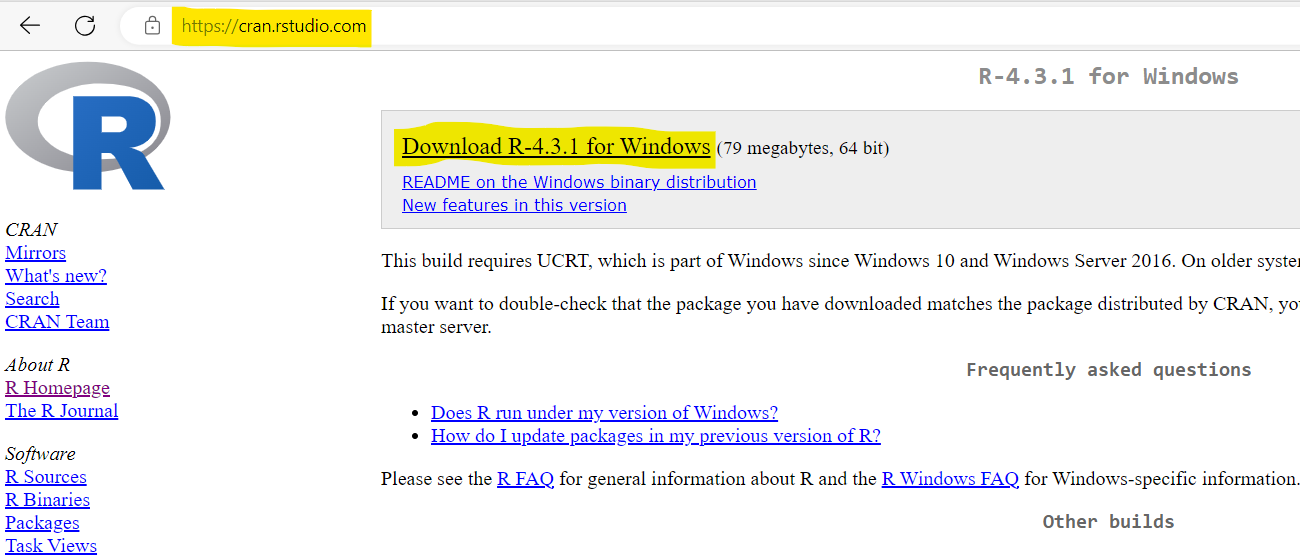(7.) Step 5:(8.) Step 6: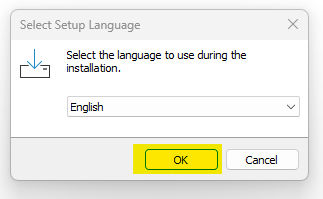(9.) Step 7:(10.) Step 8: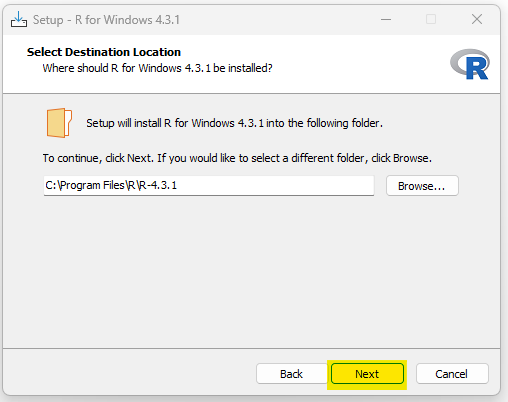(11.) Step 9: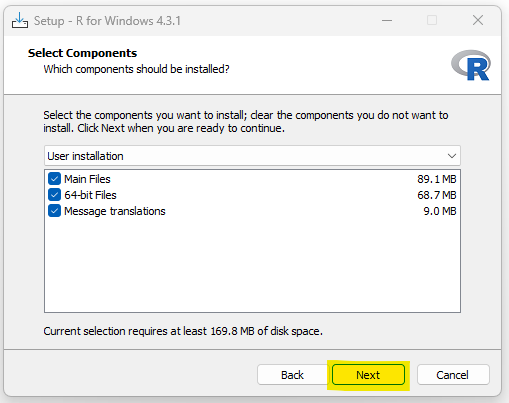(12.) Step 10: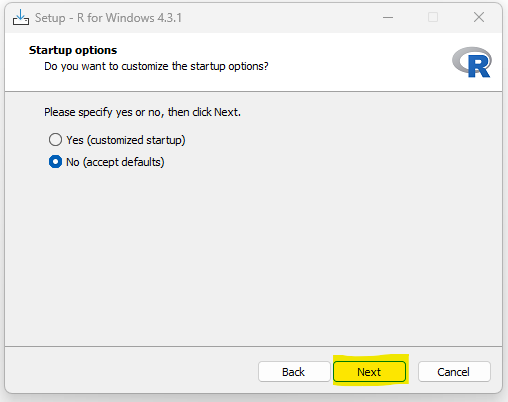(13.) Step 11: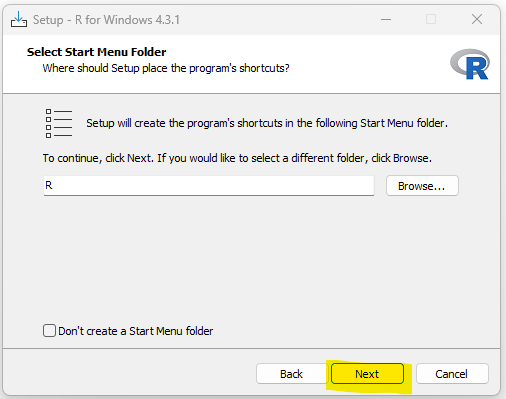(14.) Step 12: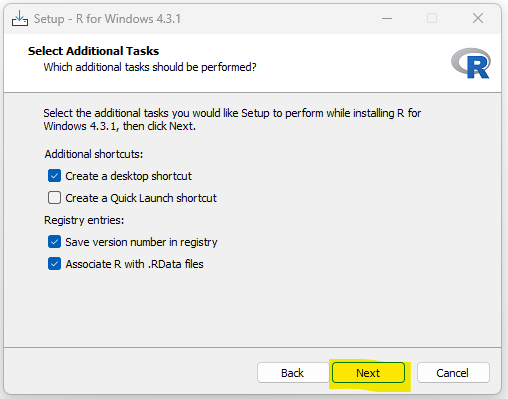(15.) Step 13: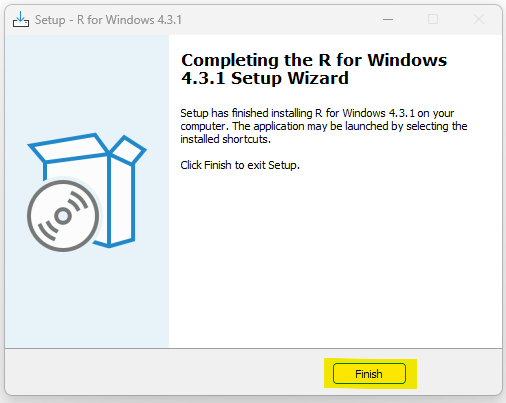As at today: 06/26/2023;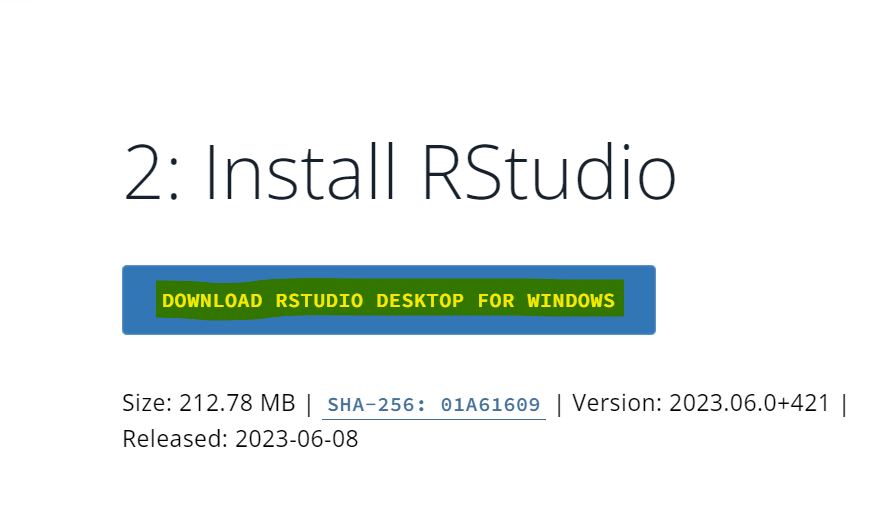(3.) Step 2: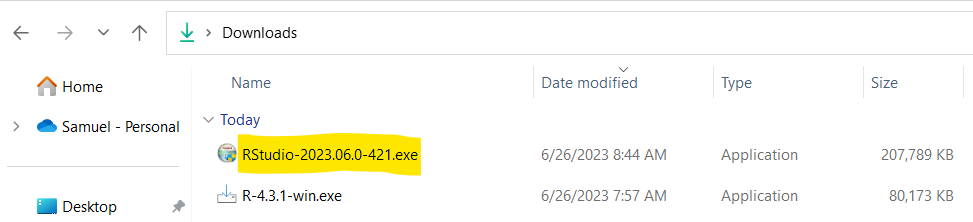(4.) Step 3: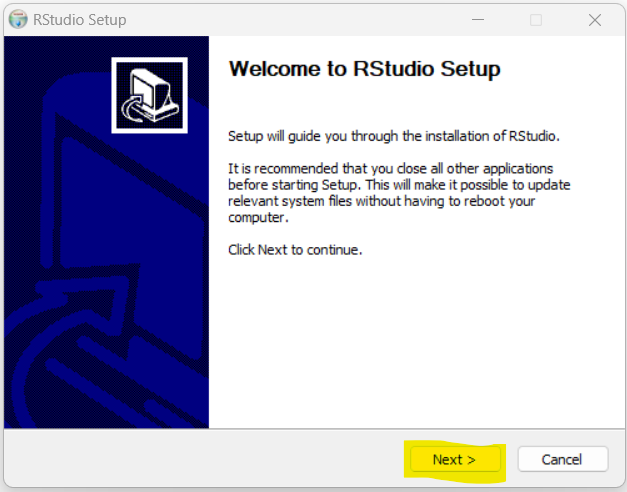(5.) Step 4:(6.) Step 5: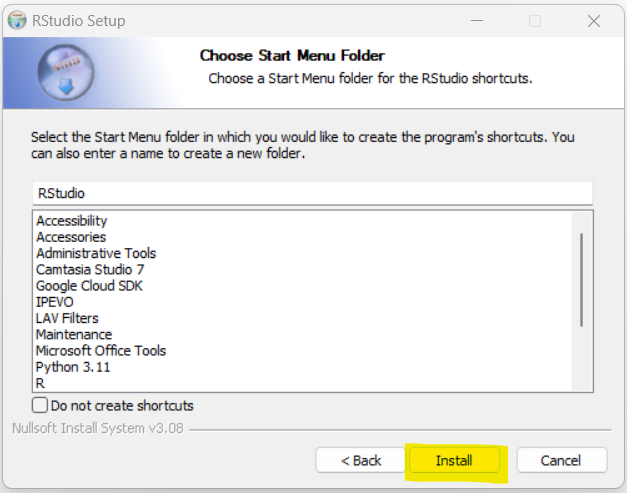(7.) Step 6: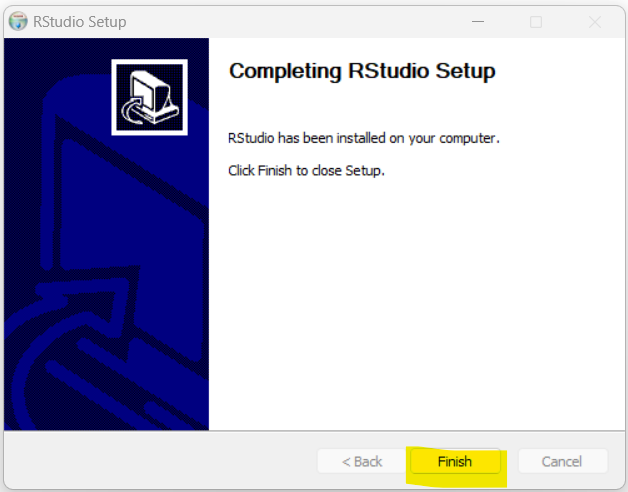## Run RStudio

Run RStudio
(1.) Step 1: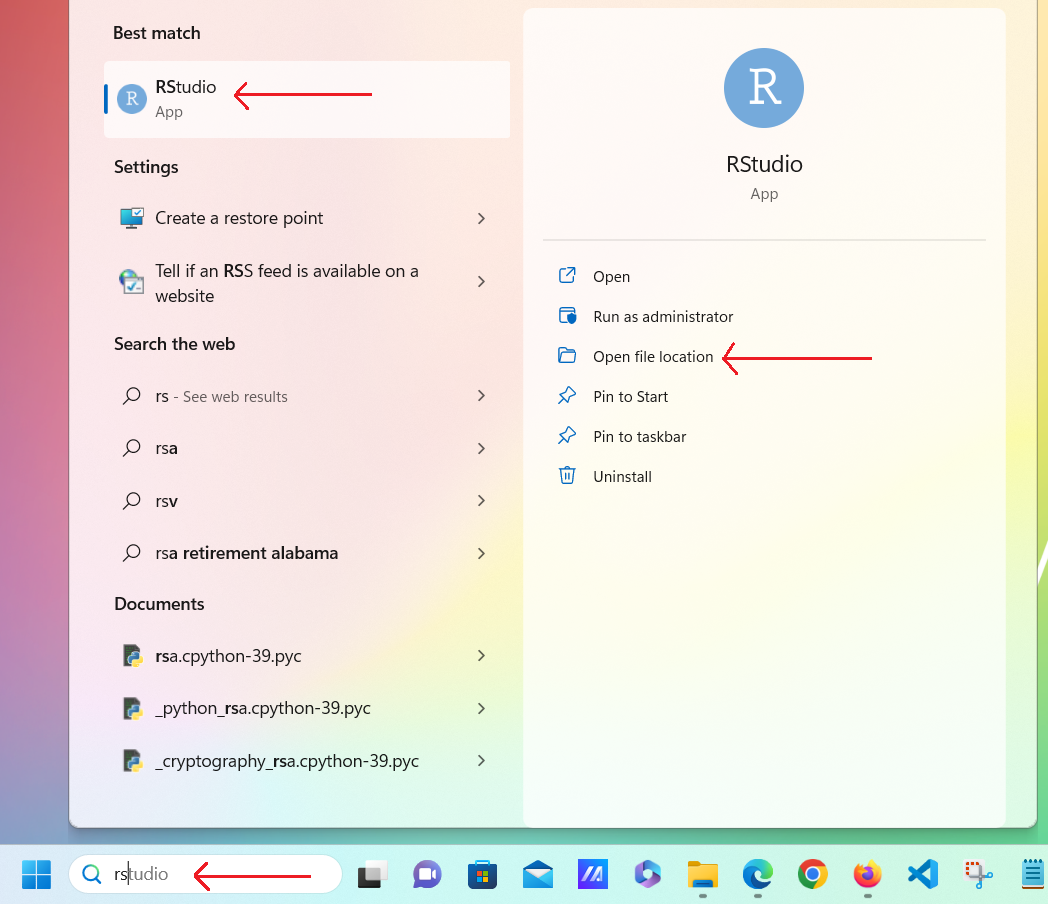(2.) Step 2: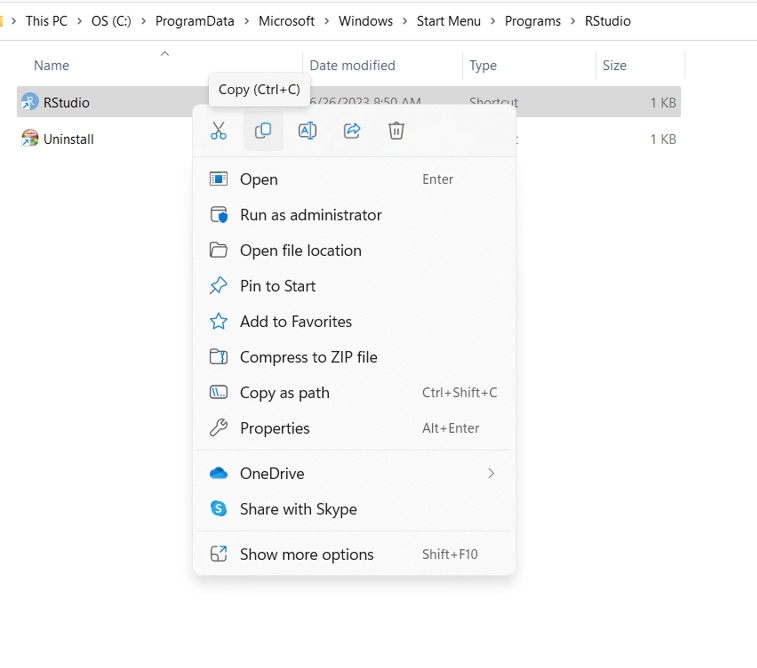(3.) Step 3: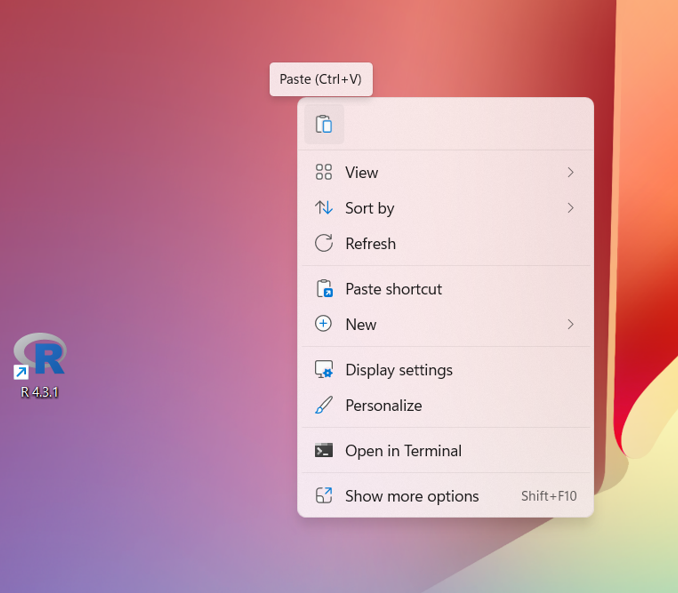(4.) Step 4: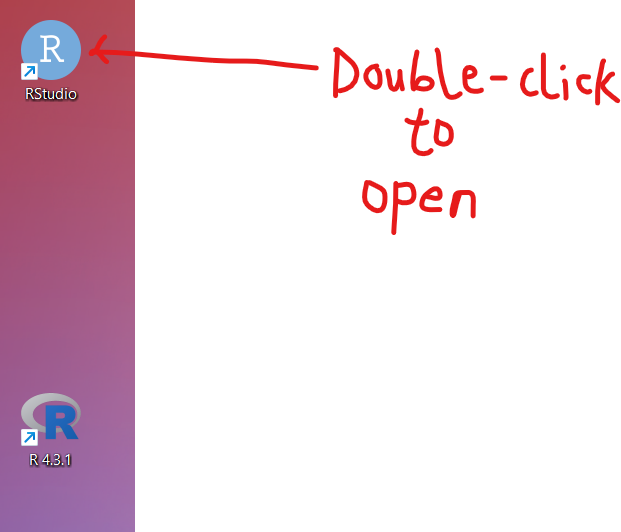(5.) Step 5: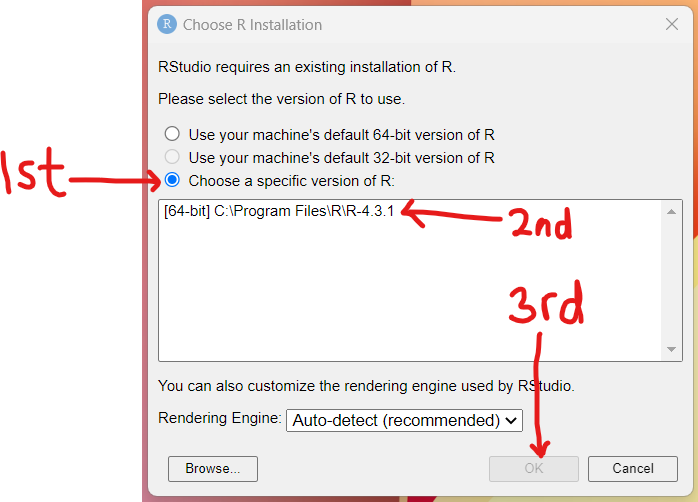(6.) Step 6: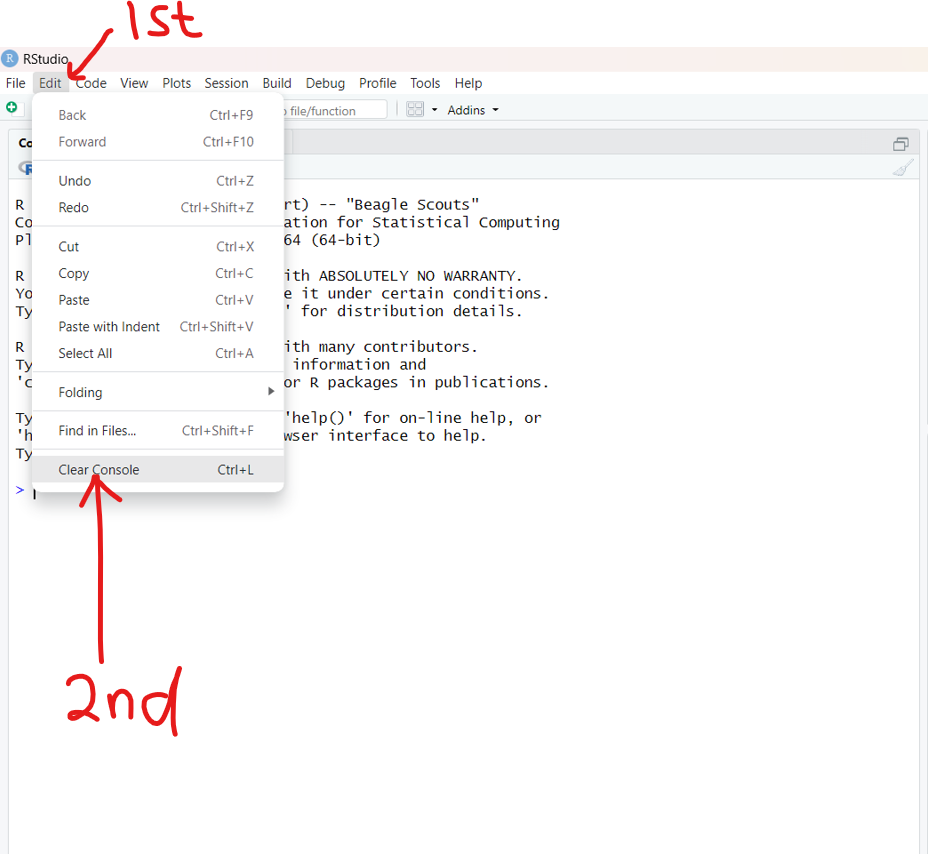It has come to my attention that some settings are necessary in RStudio.
One of the important settings is the font size of the editor. By default, it is a small font size (less than 12pt). We need to increase it.
This is to accommodate the students who may need an acceptable font size so as not to strain the eyes.
For the programs written already, if you need an acceptable font size, please attend Office Hours/Live Sessions so we can go over it.
Going forward, we shall use the acceptable font size that we want to use now.
So, let us increase the font size.

Tools menu → Global Options... bar →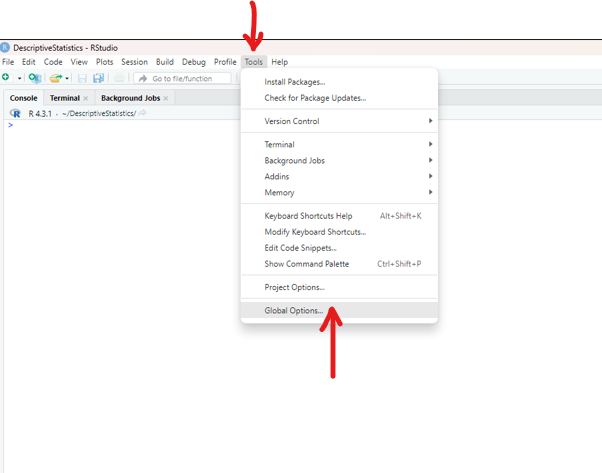Appearance → Make the appropriate settings: at least a font size of 14; ApplyOK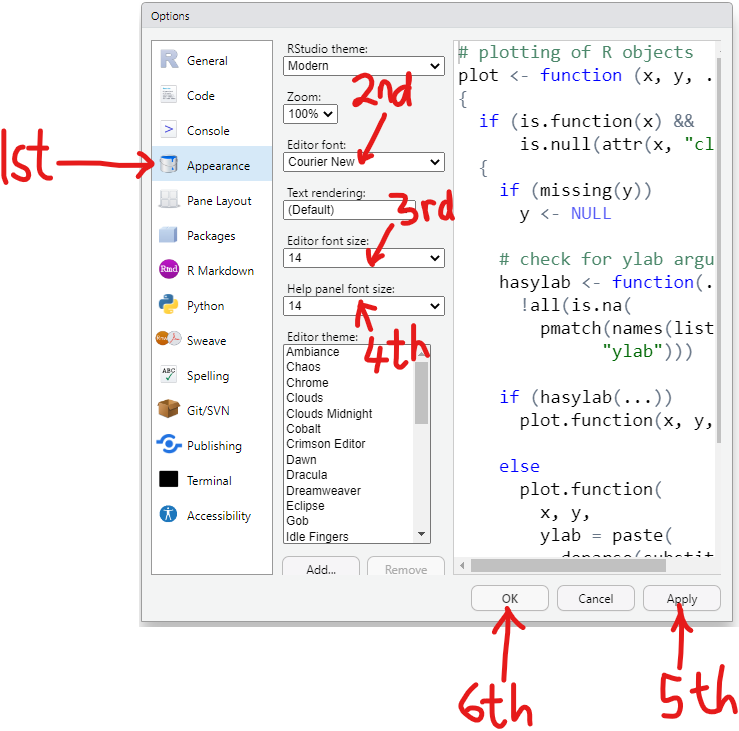## Datasets in RStudio

As at today: 06/28/2023;
To see all the datasets in RStudio:
(1.) Step 1: Type the data() or data function: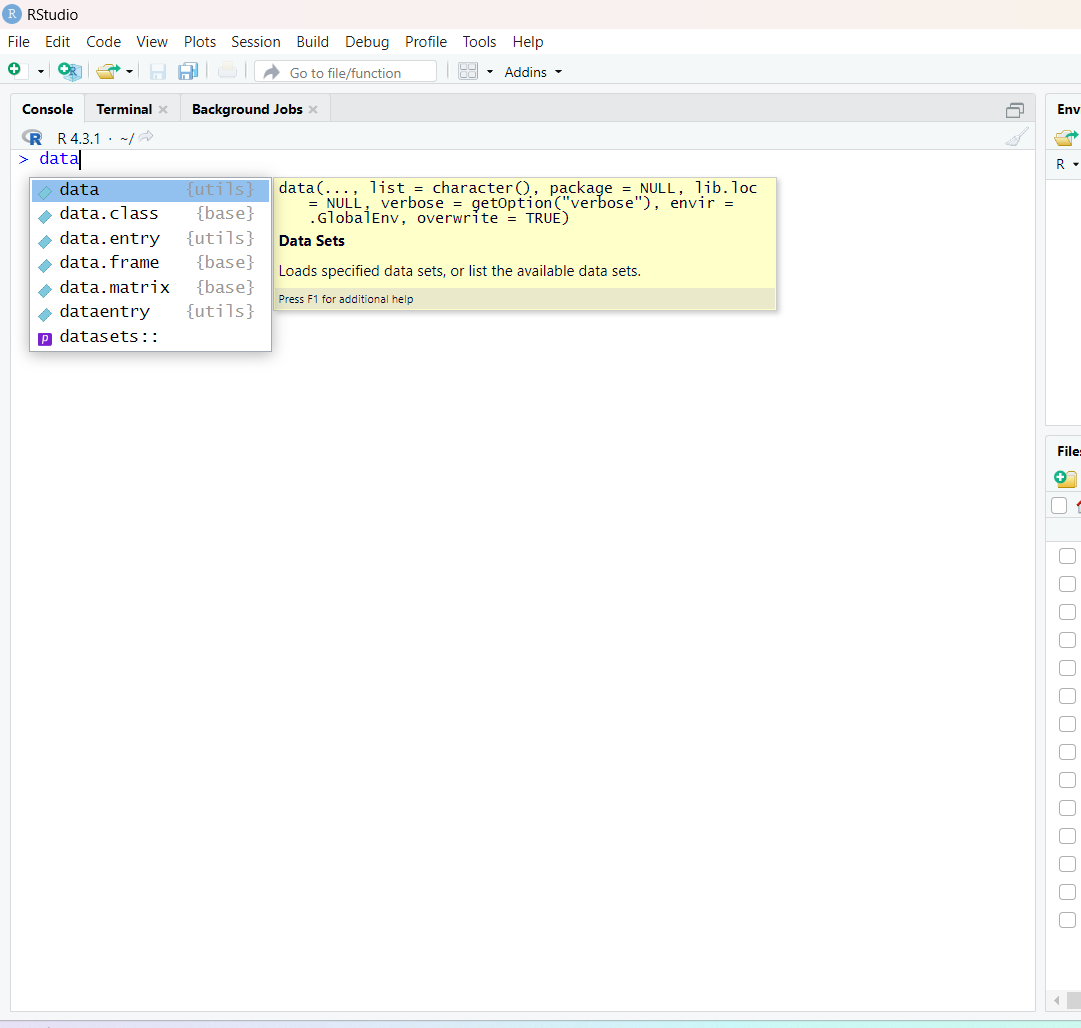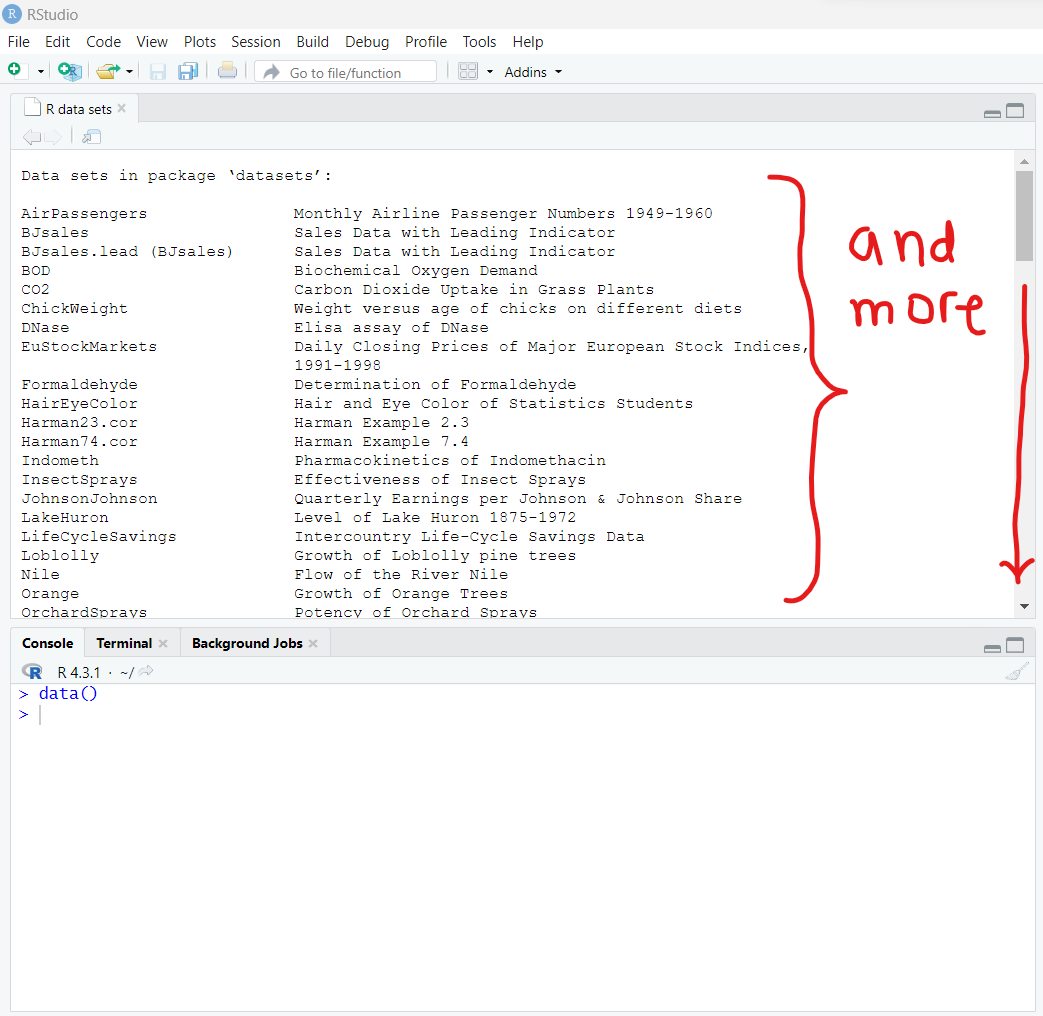Going forward, let us make comments to our code.
Comments in R are written beginning with the hatch, # symbol.

(2.) Step 2: I want a dataset with only one variable.
So, let me read the description of the rivers data so I have to use the code: ?rivers
The column is the length of the major rivers.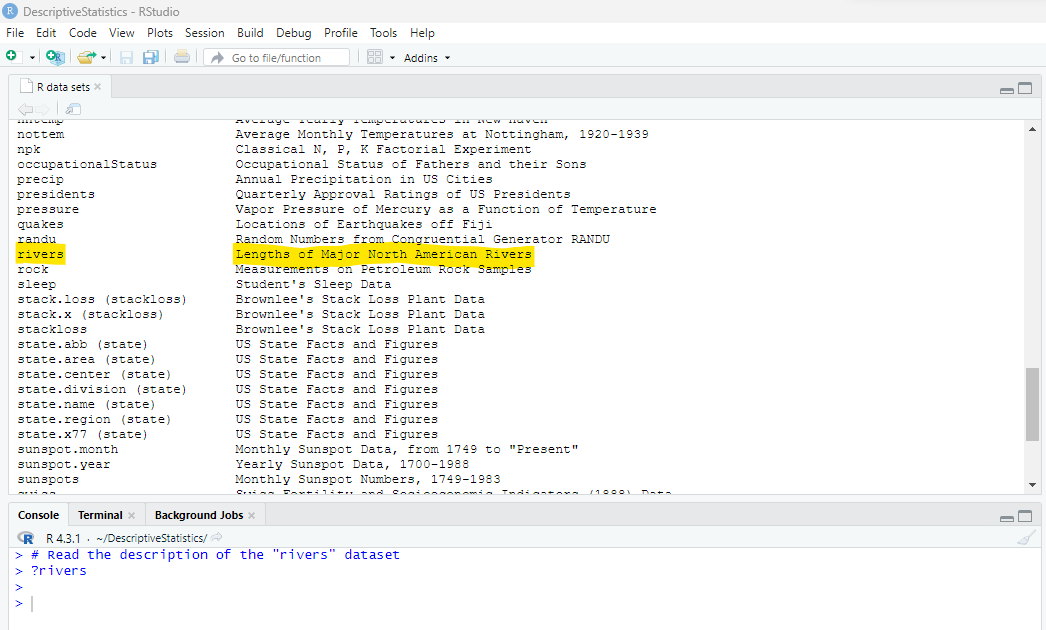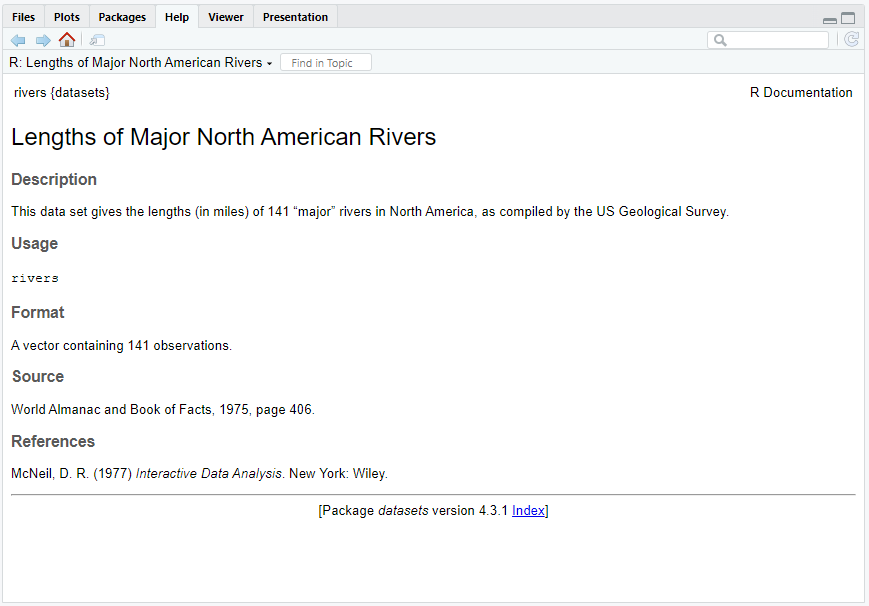(3.) The description tells me the dataset is a vector with 141 observations.
This means that the sample size is 141.
A vector is a list of items that are the same data type.
I already know that length is a quantitative: continuous variable
So, the data type must be numeric (numbers)
Let me verify the sample size by using the length() function.
Then, verify the data type of the data values by using the class() function.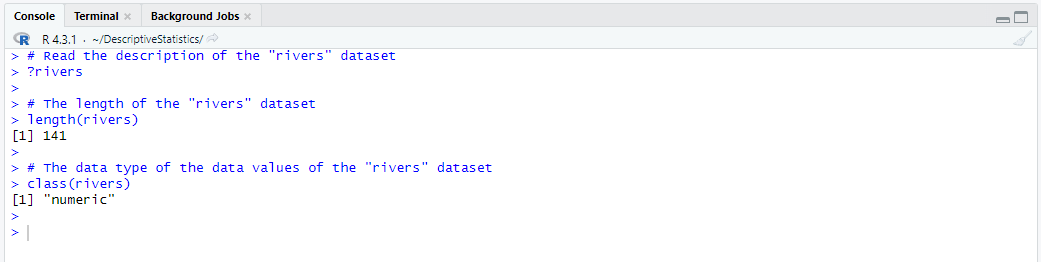(4.) I want to see the rivers data, so I have to use the code: rivers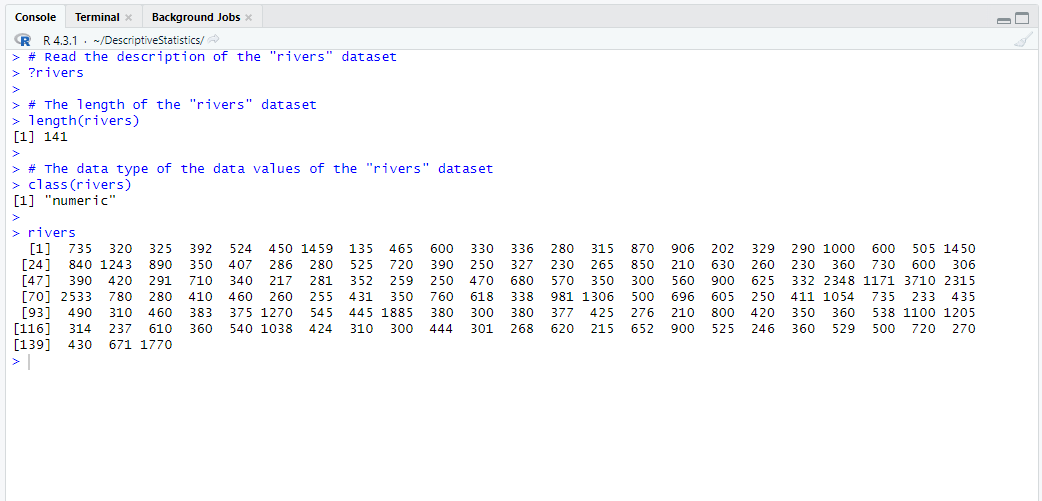(5.) I do not like the format of this dataset.
It looks like a raw data as it is.
I want it to look like a table
The name of the dataset is rivers
So, to make it look like a table, we have to convert the vector to a data frame
A data frame is data displayed in a table format.
First, let us make a copy of the dataset.
It is highly recommended that you make a copy of any built-in dataset in R that you intend to use, then use that copy.
We copy the dataset by assigning it to a new dataset using the assignment operator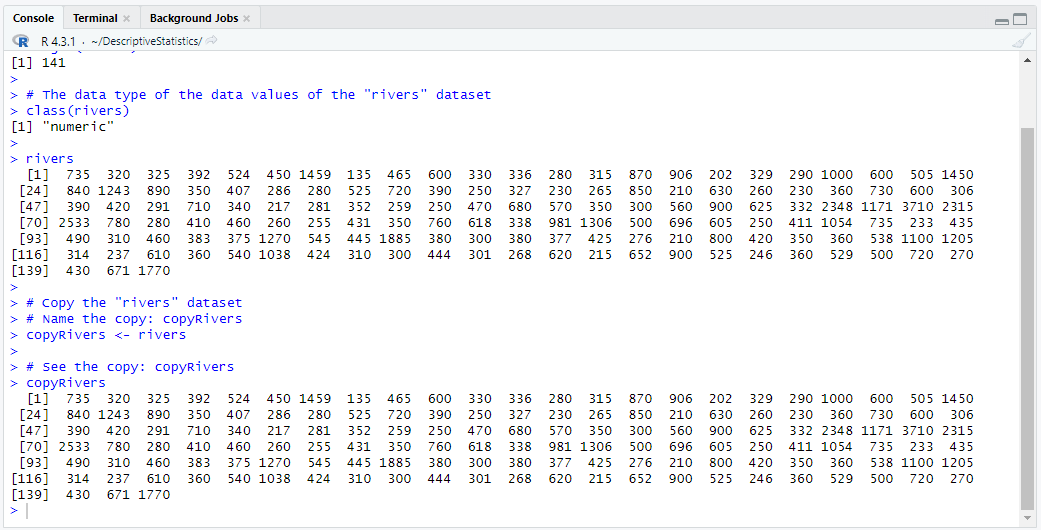Then, we can work with the copyRivers dataset to make it look like a table.
Let us do so using a new dataset and the as.data.frame() function.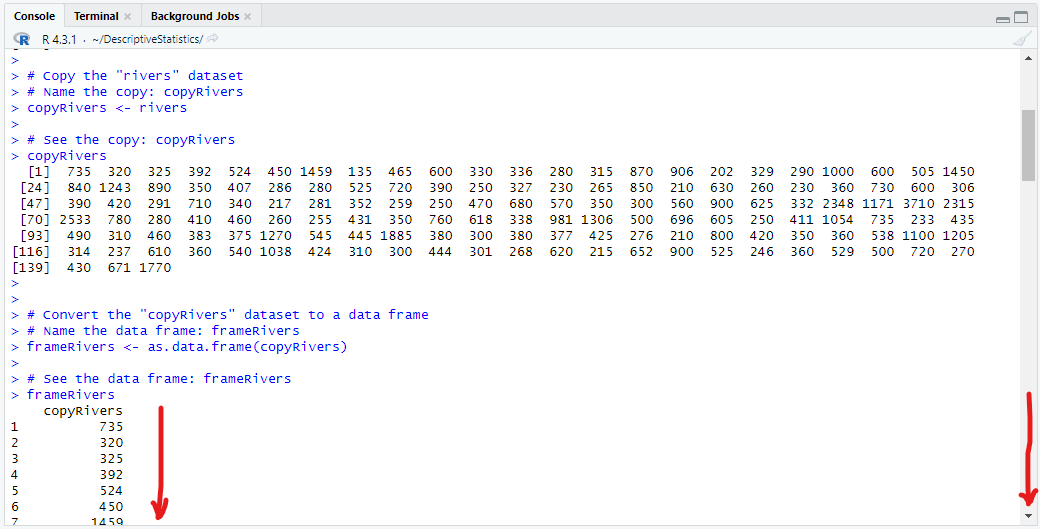Besides, the appearance, are there more differences?
Yes, there are more differences.
Say we want to find the mean of the vector and the mean of the data frame, let us observe these codes: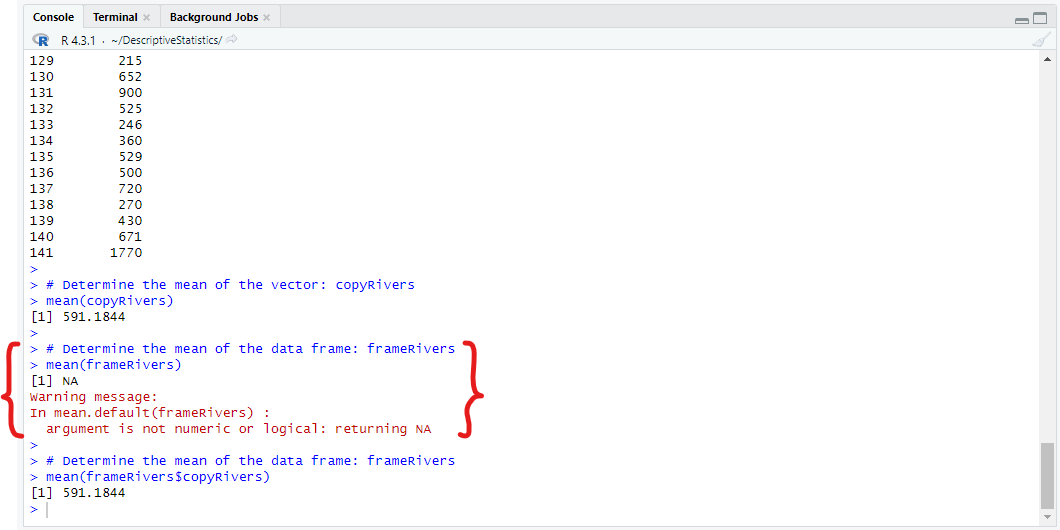Do you notice the extra step we took to determine the mean of the data frame?
As a vector, we can simply determine the mean by using the built-in mean() function directly using the vector as the argument.
As a data frame, we have to use the built-in function using the data frame and a reference (denoted by the dollar symbol, $) to the column name as the argument. So, this implies that for all numeric data type (class of the dataset is "numeric"), we can determine the built-in function directly. But for all data.frame data type (class of the dataset is "data.frame"), we have to use the data frame in reference to each variable (column name) (6.) The "rivers" dataset has only one variable: "length" So,e datasets in RStudio are data frames and have more than one column What if we want to find the mean of the entire dataset? So, let us discuss another type: the matrix. A matrix is a rectangular two-dimensional array (row and column) of data. The row are the horizontal entries (data values) The column are the vertical entries (data values) Let us review this dataset: the "women" dataset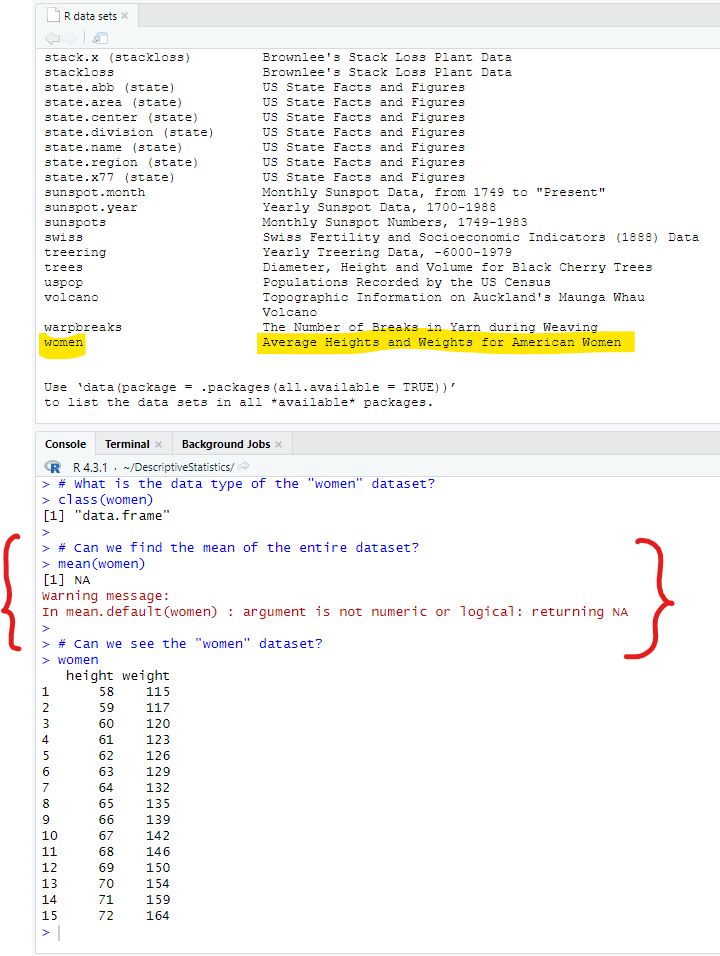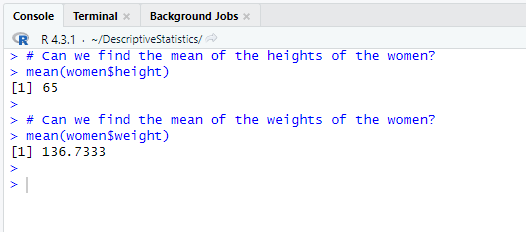But how do we find the mean of the entire dataset? One way to do so is to convert the data frame to a matrix using the as.matrix() function Then, we can find the mean of the entire dataset. So, let us make a copy and convert it to a matrix.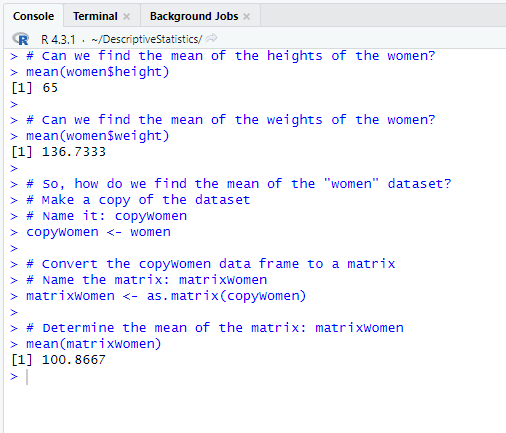There are more data types but let us discuss them if we need to. For now, let us get back to Statistics. To see more examples of using the built-in (system-defined) statistical functions and writing our user-defined functions in R/RStudio, please review R and RStudio for Descriptive Statistics ## Find, Download and Import Microsoft Excel Dataset into RStudio Let us access one of the datasets we shall be using in the course: Datasets from the U.S Government website: United States Government's Open Data: Datasets Download a dataset in Microsoft Excel format Import it into RStudio. These will require installing some packages. (1.) Step 1: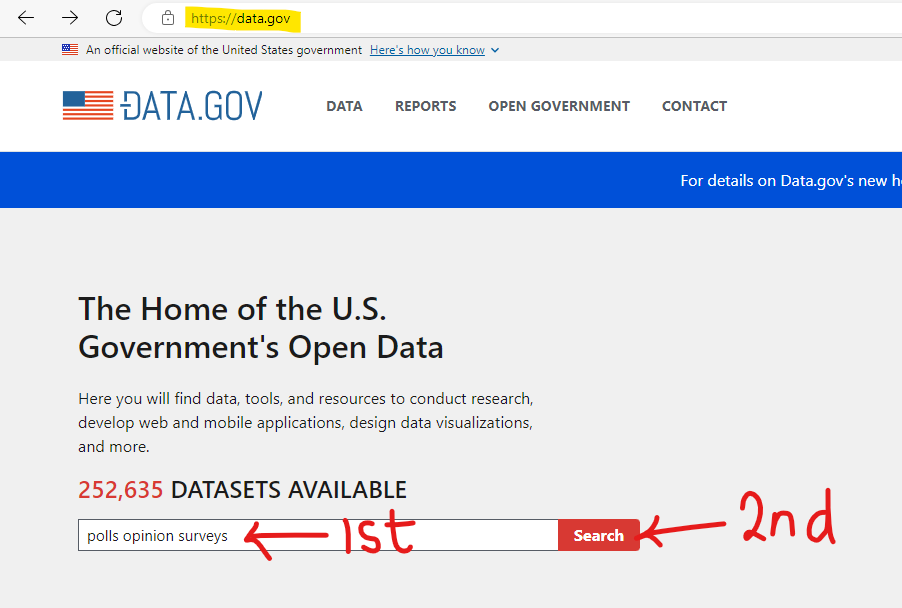(2.) Step 2: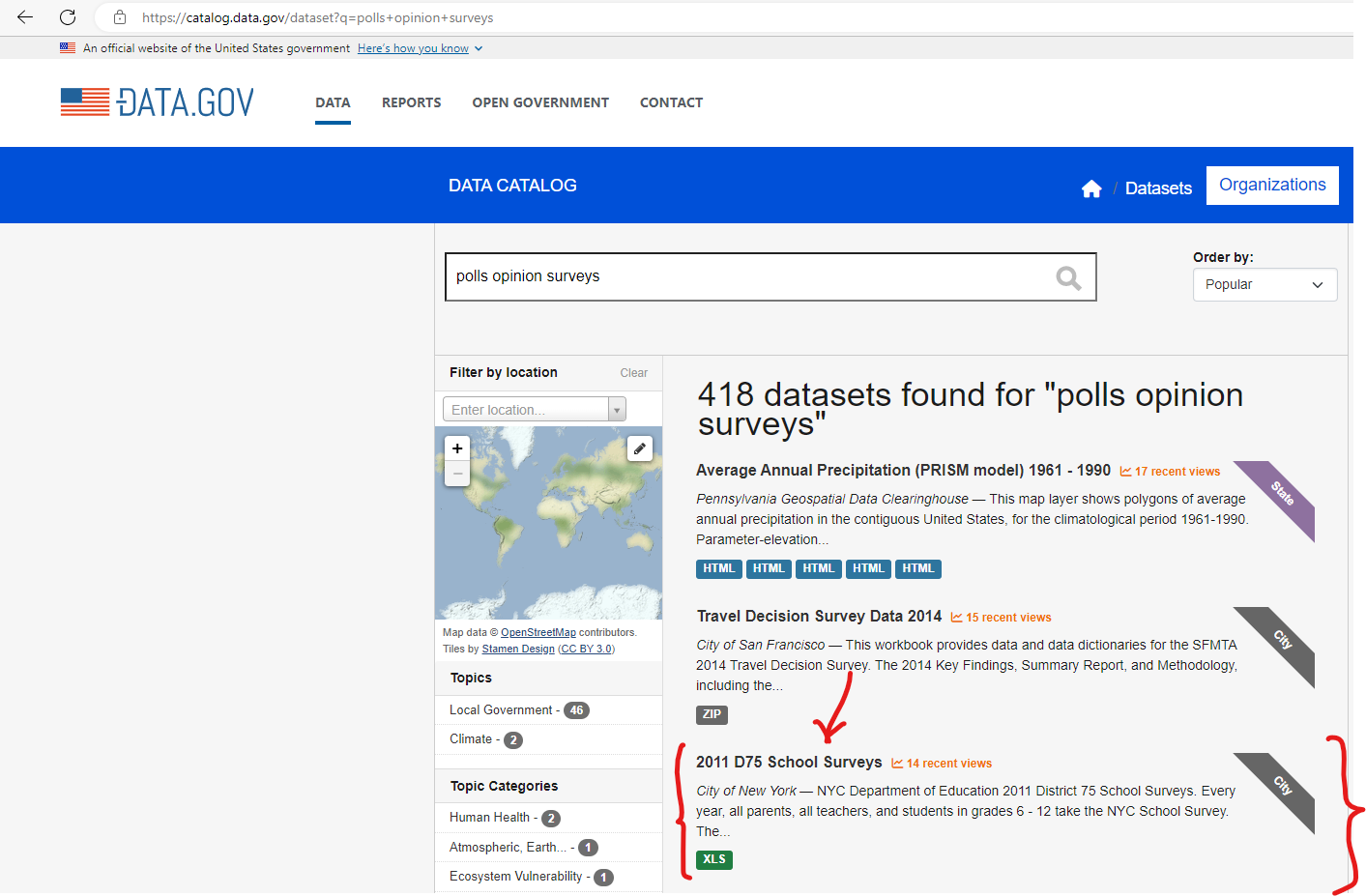(3.) Step 3: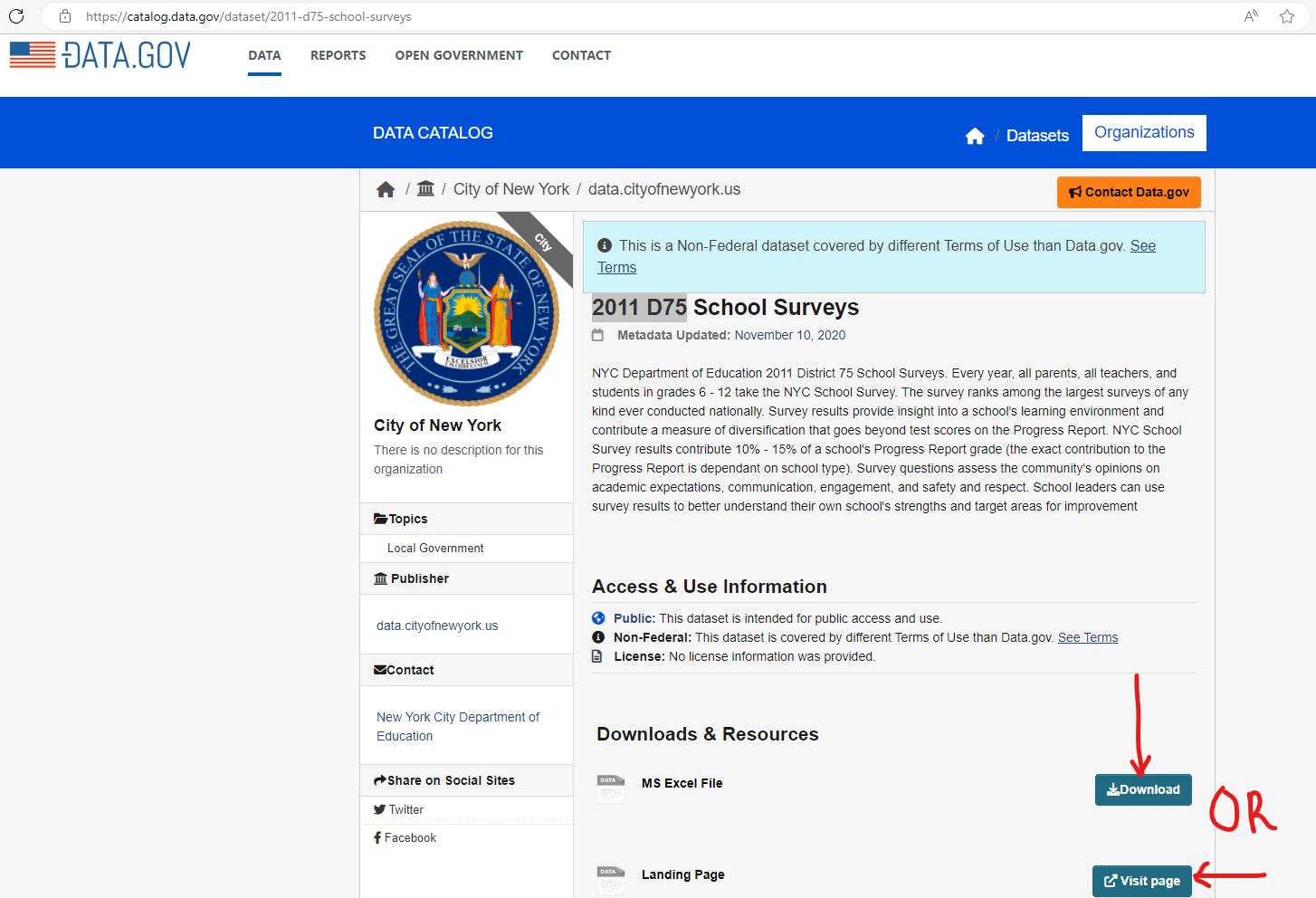(4.) Step 4:(5.) Step 5:(6.) Step 6: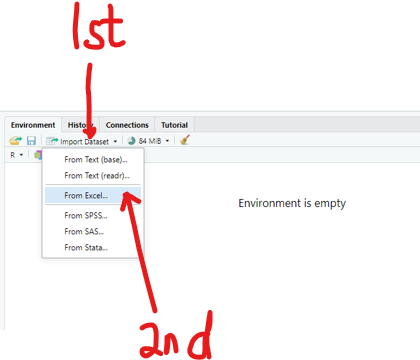(7.) Step 7: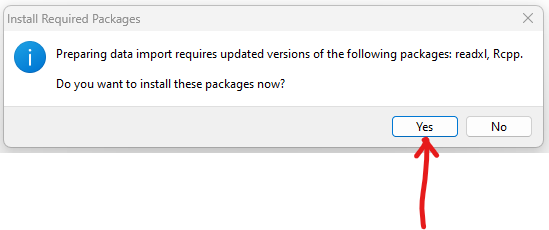(8.) Step 8: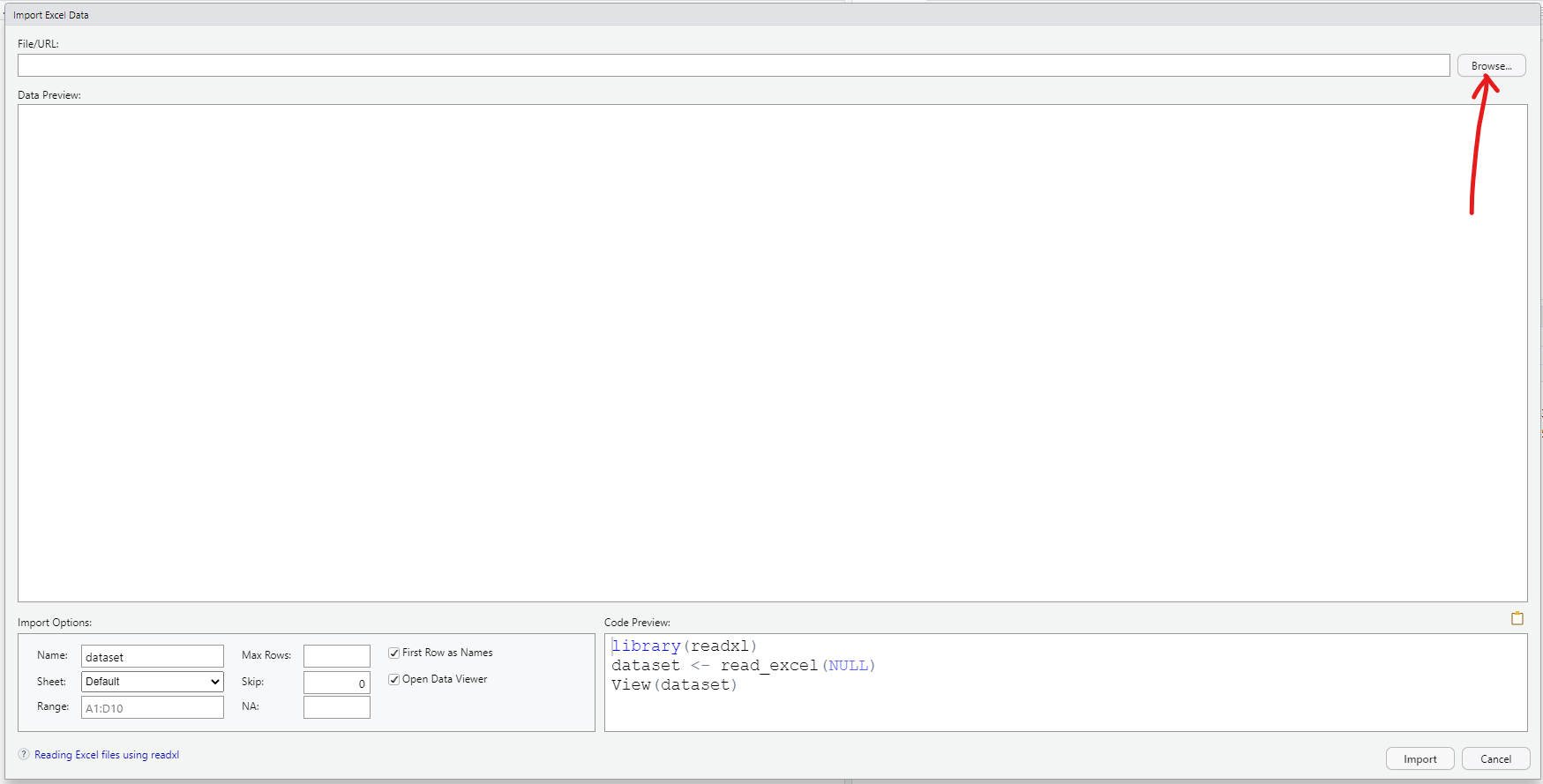(9.) Step 9: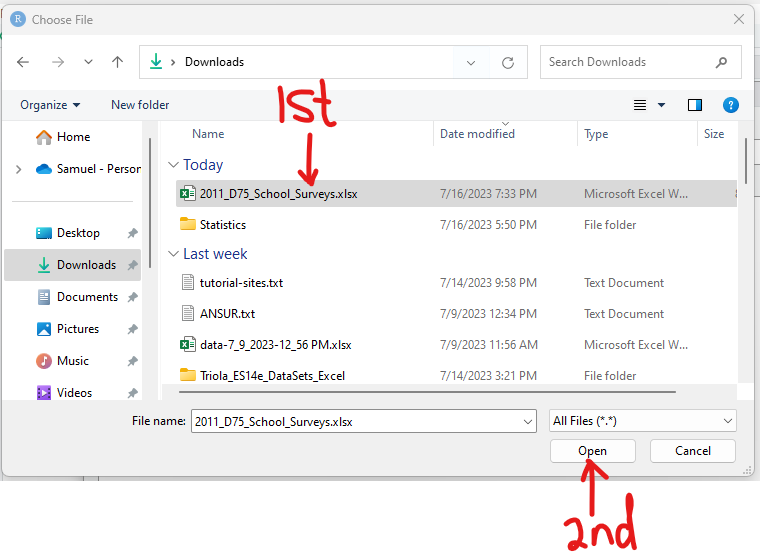(10.) Step 10: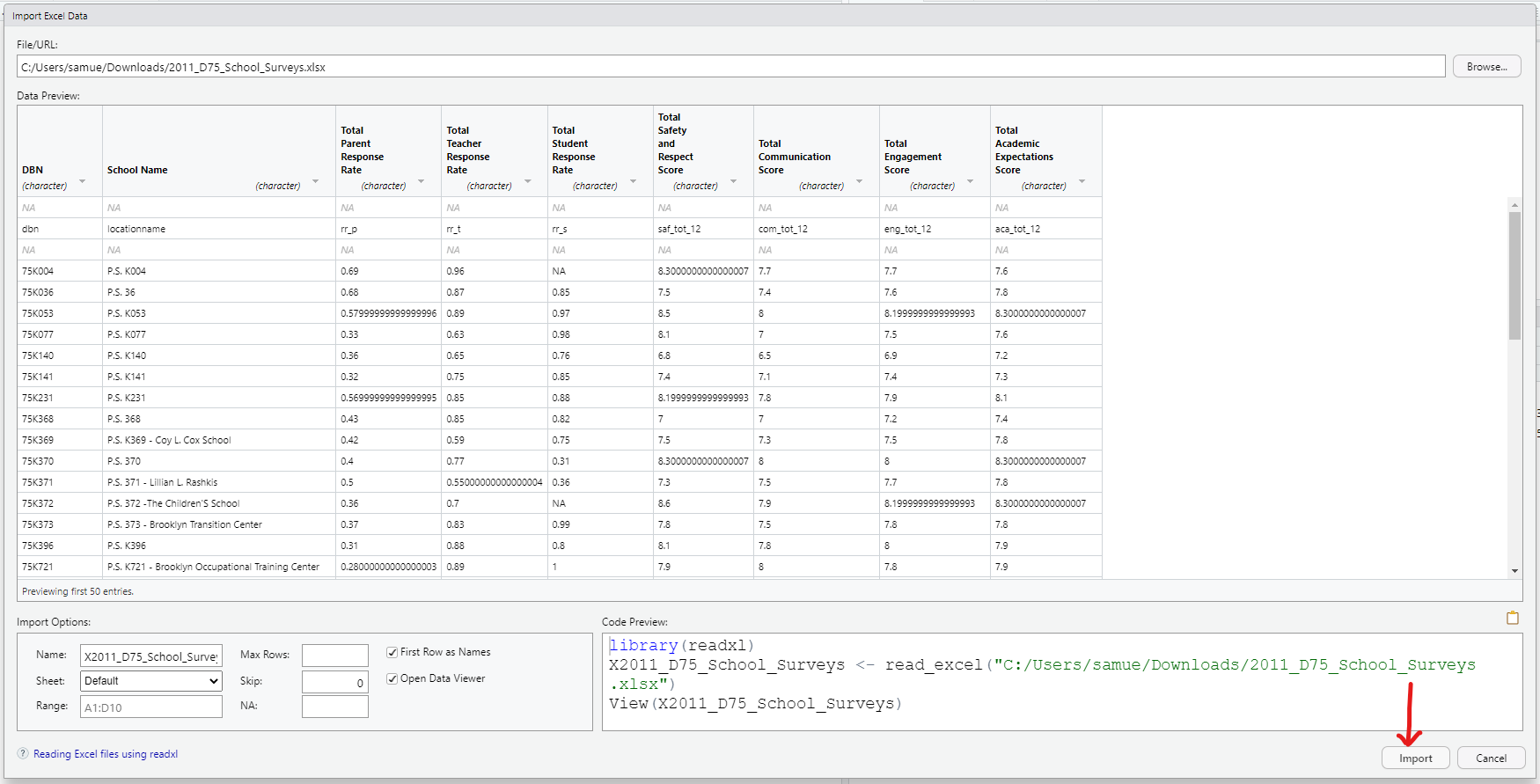(11.) Step 11: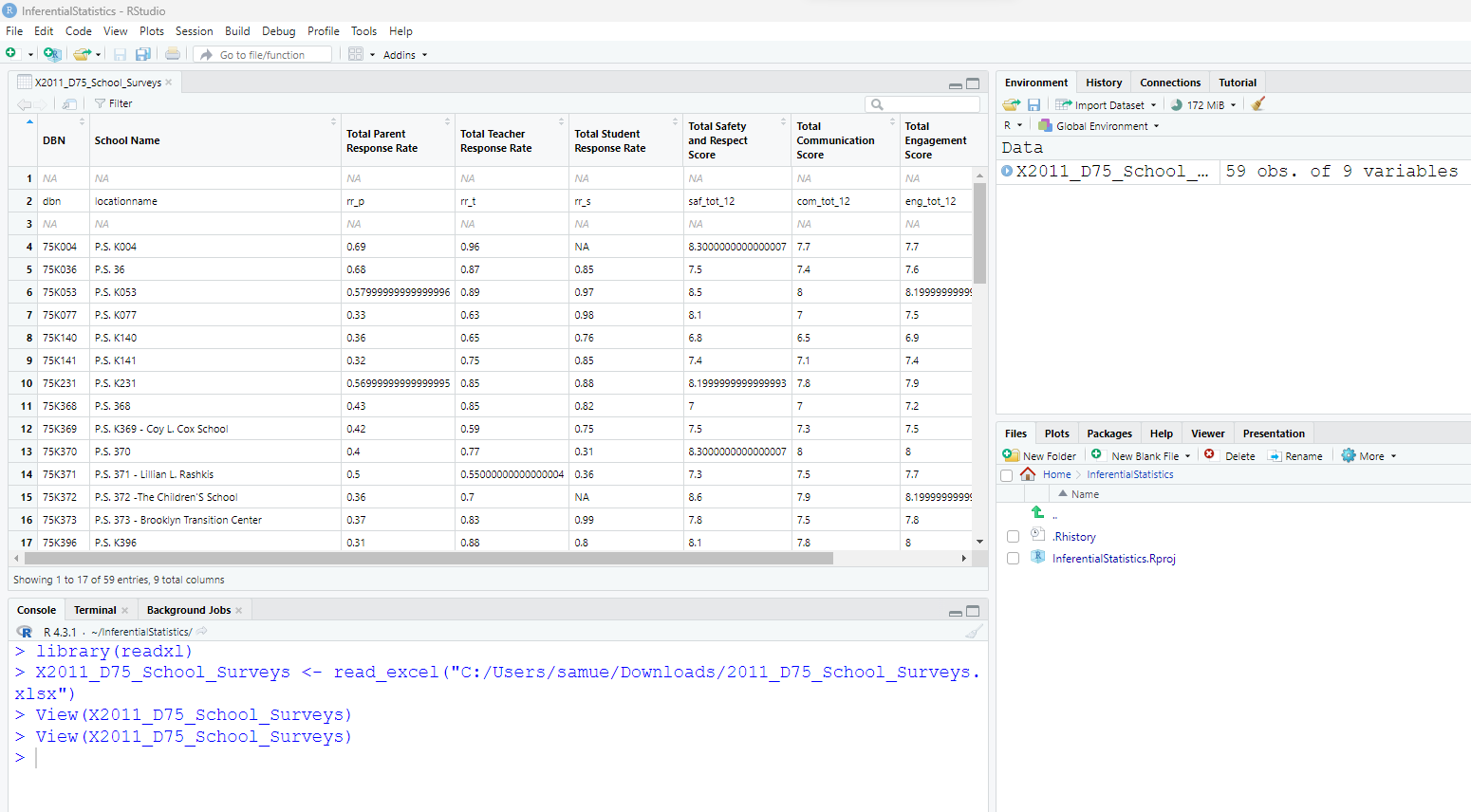## Variables A Variable is a portion of computer memory for storing a data value. It is created by assigning a value or an expression to it. Assigning a value to a variable is done with the assignment operator:$\lt$– An equal sign, = is used in some cases; however the assignment operator is highly recommended. It is also highly recommended that: (A.) The variable is on the LHS (Left Hand Side) of the assignment operator. (B.) The value or the expression is on the RHS (Right Hand Side) of the assignment operator. For example, we declare a variable in R this way:  variable <– value  OR  variable <– expression  Variable names: (1.) Can be single letters (please avoid unless if used in loops) (2.) (a.) Can contain a combination of letters, digits, and underscores but must not begin with a digit. (b.) It may begin with a letter or a period (not an underscore). (c.) If it starts with a period, it cannot be followed by a digit. (d.) Also, it should not contain two consecutive underscores. Be it as it may: (a.) Please avoid underscores and decimals (b.) Use a combination of letters and digits such as: num1 to represent the first number variable1 to represent the first number (3.) Can contain only letters but must not be any of the keywords or system-defined functions. It is highly recommended to avoid using any keywords as an identifier in any R program. The keywords are: if, else, repeat, while, function, for, next, break, TRUE, FALSE, NULL, Inf, NaN, NA, NA_integer_, NA_real_, NA_complex_, NA_character_, The functions are: (a.) The letters can be Camel case (similar to the hump of a camel) such as: firstVariable...the V is the hump In Camel casing letters: two letters are merged as one; the first letter of the second word is an uppercase letter while all other letters are lower case letters. In firstVariable, V is uppercase; all other letters are lower case (b.) The letters can be Pascal case such as FirstVariable In Pascal casing, two letters are merged as one; the first letter of the first word and the first letter of the second word are uppercase letters while all other letters are lower case letters. In FirstVariable, F and V are uppercase; all other letters are lowercase. (c.) uppercase letters (please avoid...it denotes someone who is yelling) (d.) lowercase letters (okay, but try to avoid) Student: What if you have three words? How do you write it in Camel case? Pascal case? Teacher: Say we want to write the variable, first arithmetic sequence Camel case: firstArithmeticSequence...A and S are the only uppercase Pascal case: FirstArithmeticSequence...F, A, S are the only uppercase In this course, for all applicable variables; please use: (i.) A combination of letters and digits such as num1 OR (ii.) Camel case letters such as firstNumber OR (iii.) Pascal case letters such as FirstNumber ***We shall use single characters when we write conditions for Iteration statements/Loops because it is easier to use single characters in such cases. We shall see examples when we discuss loops. However, feel free not to use it if you wish.*** (4.) Cannot contain whitespaces. A whitespace is a horizontal or vertical space. For example: myNum has no whitespace; but my Num has a whitespace. Variable names cannot contain whitespaces. Hence: myNum is acceptable, but my Num is not acceptable. It is also highly recommended to avoid special characters in C# variable names. Special characters are all the non-numeric and non-alphabet characters on your keyboard such as ~ (tilde), !(exclamation), @ (asperand), # (hash),$ (dollar), % (percent), ^ (caret), & (ampersand), * (asterisk).
For all course work, please avoid special characters in variable names.

(5.) Are case sensitive.
These are all different variables:
firstresult (lowercase),
firstResult (Camel case),
FirstResult (Pascal case),
Firstresult,
FIRSTRESULT (uppercase),
first_result (snake case)
strFirstResult (Hungarian notation)

Student: Hungarian notation?
Teacher: Hungarian notation is a naming convention that follows the camel case naming but in which the name is preceded by the data type represented by a three-character ID.
str means string
Similarly, int is for integer; dec is for decimal; dbl is for double; bln is for Boolean among others.